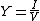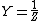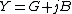Also found in: Dictionary, Medical, Legal.

Electrical engineering the reciprocal of impedance, usually measured in siemens. It can be expressed as a complex quantity, the real part of which is the conductance and the imaginary part the susceptance

The ratio of the current to the voltage in an alternating-current circuit. In terms of complex current I and voltage V, the admittance of a circuit is given by Eq. (1), and is related to the impedance of the circuit Z by Eq. (2). Y is a complex number given by Eq. (3).

(1)(2)(3)G, the real part of the admittance, is the conductance of the circuit, and B, the imaginary part of the admittance, is the susceptance of the circuit. The units of admittance are called siemens or mhos (reciprocal ohms). See Conductance, Susceptance